Documentation

## Perform Safe Trajectory Tracking Control Using Robotics Manipulator Blocks

This example shows you how to use Simulink® with Robotics System Toolbox™ manipulator algorithm blocks to achieve safe trajectory tracking control for a simulated robot running in Simscape™ Multibody™.

Both Robotics System Toolbox and Simscape Multibody are required to run this example.

### Introduction

In this example, you will run a model that implements a computed-torque controller with joint position and velocity feedback using manipulator algorithm blocks. The controller receives joint position and velocity information from a simulated robot (implemented using Simscape Multibody) and sends torque commands to drive the robot along a given joint trajectory. A planar object is placed in front of the robot so that the end effector of the robot arm will collide with it when the trajectory tracking control is executed. Without any additional setup, the increasing torque due to colliding with the object can cause damage on the robot or the object. To achieve safe trajectory tracking, a trajectory scaling block is built to adjust the time stamp when assigning the desired motion to the controller. You may adjust some parameters and interact with the robot while the model is running and observe the effect on the simulated robot.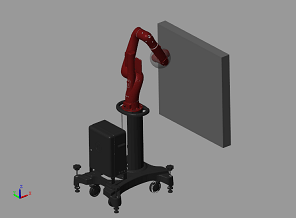### Set Up the Robot Model in Workspace

This example uses a model of the Rethink Sawyer, a 7 degree-of-freedom robot manipulator. Call `importrobot` to generate a `rigidBodyTree` model from a description stored in a Unified Robot Description Format (URDF) file. Set the `DataFormat` and `Gravity` properties to be consistent with Simscape.

```sawyer = importrobot('sawyer.urdf'); sawyer.removeBody('head'); sawyer.DataFormat = 'column'; sawyer.Gravity = [0, 0, -9.80665];```

### Trajectory Generation and Related Setup

First, assign the start time and duration for the trajectory.

```tStart = 0.5; tDuration = 3;```

Next, assign the initial and target configuration. `q0` is the initial configuration and `q1` is the target configuration.

```q0 = [0; -1.18; 0; 2.18; 0; -1.0008; 3.3161]; q1 = zeros(7,1);```

The following figures show the robot visualization of the initial configuration and the target configuration related to the location of the planar object. The planar object is placed so that the robot will collide to it during trajectory tracking.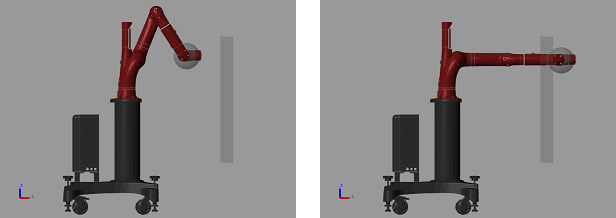Use `exampleHelperPolynomialTrajectory` to generate the desired motion trajectory for each joint. `exampleHelperPolynomialTrajectory` generates the polynomial coefficients of a trajectory that satisfies the desired joint position, zero velocity and zero acceleration based on the initial and target configurations and the trajectory duration.

`p = exampleHelperPolynomialTrajectory(q0,q1,tDuration);`

### Simulink Model Overview

Next, open the Simulink model. The variables generated above are already stored in Simulink model workspace:

`open_system('robotSafeTrajectoryTracking.slx');`

The `robotSafeTrajectoryTracking` model implements a computed torque controller with trajectory scaling for safe trajectory tracking. There are three main subsystems in this model:

• Computed Torque Controller

• Trajectory Scaling and Desired Motion

• Simscape Multibody Model with Simple Contact Mechanics

On each time step, if the trajectory scaling switch is on, the modified time stamp is used for evaluating the desired joint position, velocity and acceleration. Then, the computed torque controller uses the manipulator blocks associated with the `RigidBodyTree` model to track the desired motion. The derived control input is fed into the Sawyer model in Simscape Multibody (where the planar object for interacting with the robot is included).

### Build Computed Torque Controller Using Robotics Manipulator Blocks

For a manipulator with $n$ non-fixed joints, the system dynamics can be expressed as:

`$M\left(q\right)\underset{}{\overset{¨}{q}}+C\left(q,\underset{}{\overset{˙}{q}}\right)\underset{}{\overset{˙}{q}}+G\left(q\right)=u$`

where $q$, $\underset{}{\overset{˙}{q}}$, $\underset{}{\overset{¨}{q}}$, $\in {R}^{n}$ are the position, velocity and acceleration of the generalized coordinate, $u\in {R}^{n}$ is the control input (torque), $M\left(q\right)$ is the joint space mass matrix, $C\left(q,\underset{}{\overset{˙}{q}}\right)\underset{}{\overset{˙}{q}}$ is the velocity product torque, $G\left(q\right)$ is the gravity torque. To track along a desired joint trajectory with desired position ${q}_{d}$, velocity ${\underset{}{\overset{˙}{q}}}_{d}$ and acceleration ${\underset{}{\overset{¨}{q}}}_{d}$, the computed torque controller calculates the torque needed to obtain a given configuration and velocity, provided the robot dynamics variables $M\left(q\right)$, $C\left(q,\underset{}{\overset{˙}{q}}\right)\underset{}{\overset{˙}{q}}$, and $G\left(q\right)$. In Simulink, these variables can be easily derived using robotics manipulator blocks from Robotics System Toolbox to design the following computed torque controller:

`$u=M\left(q\right)\left({\underset{}{\overset{¨}{q}}}_{d}-{K}_{d}{\underset{}{\overset{˙}{q}}}_{e}-{K}_{p}{q}_{e}\right)+C\left(q,\underset{}{\overset{˙}{q}}\right)\underset{}{\overset{˙}{q}}+G\left(q\right)$`

where ${q}_{e}=q-{q}_{d}$ is the position error and ${\underset{}{\overset{˙}{q}}}_{e}=\underset{}{\overset{˙}{q}}-{\underset{}{\overset{˙}{q}}}_{d}$ is the velocity error. With this controller input, the system dynamics becomes a second-order ODE:

`${\underset{}{\overset{¨}{q}}}_{e}+{K}_{d}{\underset{}{\overset{˙}{q}}}_{e}+{K}_{p}{q}_{e}=0$`

By choosing ${K}_{d}$ and ${K}_{p}$ properly, the tracking error ${q}_{e}$ will converge to zero when time approaches infinity.

The Computed Torque Controller subsystem is built using three robotics manipulator blocks: Joint Space Mass Matrix, Velocity Product Torque, and Gravity Torque. Note that the associated `rigidBodyTree` model, `sawyer`, is assigned in all those blocks, and the configuration and velocity need to be inserted as column vectors.

`open_system('robotSafeTrajectoryTracking/Computed Torque Controller');`

Inside the Computed Torque Controller, there are two tunable parameters (indicated by colored blocks):

• Gain `Kp`: The proportional gain when correcting the robot configuration

• Gain `Kd`: The derivative gain when correcting the robot configuration

A standard way to determine the `Kp` and `Kd` is to calculate them as:

`${K}_{p}={\omega }_{n}^{2}$`

`${K}_{d}=2{\omega }_{n}\xi$`

where ${\omega }_{n}$ and $\xi$ are the natural frequency and damping ratio of the second-order ODE. In this example, the default value of `Kp` and `Kd` are derived by setting the natural frequency and damping ratio as ${\omega }_{n}=10$ and $\xi =1$ to make the second-order ODE a critical damped system.

### Trajectory Scaling

There are two main blocks in this subsystem:

• Trajectory Scaling

• Desired Motion

Trajectory Scaling is the main block deployed for safe trajectory tracking in this example. At each time step ${t}_{i}$, the original time stamp is calculated as ${t}_{i}={t}_{i-1}+\Delta t$. However, when the robot collides with an unexpected object, the increasing torque and deviance from the planned trajectory can be destructive for both the robot and the object. The main idea of trajectory scaling is to calculate the time stamp as ${t}_{i}={t}_{i-1}+{f}_{s}\left({q}_{d},{\underset{}{\overset{˙}{q}}}_{d},{\underset{}{\overset{¨}{q}}}_{d},{\tau }_{mea}\right)\Delta t$ by introducing ${f}_{s}\left({q}_{d},{\underset{}{\overset{˙}{q}}}_{d},{\underset{}{\overset{¨}{q}}}_{d},{\tau }_{mea}\right)\in \left[-1,1\right]$, which is a function of the desired motion and measured torque ${\tau }_{mea}$. The function ${f}_{s}\left(\right)$ controls the speed of the robot motion and is determined based on the interference felt by the robot. If the measured torques are greater than expected, ${f}_{s}\left(\right)$ is decreased to make the robot slow down or even move backward until the desired torques are achieved. These values of ${f}_{s}\left(\right)$ have the following effects on the robot's motion:

• ${f}_{s}\left(\right)>0$, the robot moves forward (${f}_{s}\left(\right)=1$ is the normal speed).

• ${f}_{s}\left(\right)=0$, the robot stops.

• ${f}_{s}\left(\right)<0$, the robot moves backward.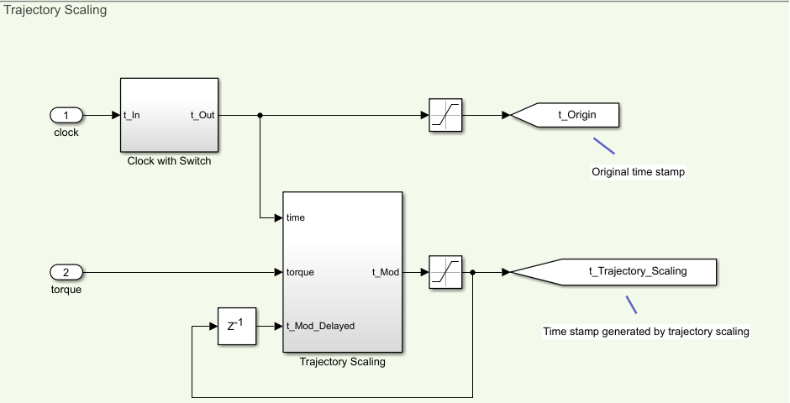In the Trajectory Scaling block, it is required to estimate the external torque ${\underset{}{\overset{ˆ}{\tau }}}_{ext}={\tau }_{mea}-\underset{}{\overset{ˆ}{\tau }}$ to calculate ${f}_{s}\left(\right)$, where ${\tau }_{mea}$ is the measured torque from the Simscape model, and $\underset{}{\overset{ˆ}{\tau }}$ is the expected torque of the desired motion on the previous time stamp. In the External Torque Observer section of the model, the Inverse Dynamics block calculates the expected torque which is subtracted from the measure torque. Expected torque is: $\underset{}{\overset{ˆ}{\tau }}=M\left({q}_{d}\right)\left({\underset{}{\overset{¨}{q}}}_{d}\right)+C\left({q}_{d},{\underset{}{\overset{˙}{q}}}_{d}\right){\underset{}{\overset{˙}{q}}}_{d}+G\left({q}_{d}\right)$.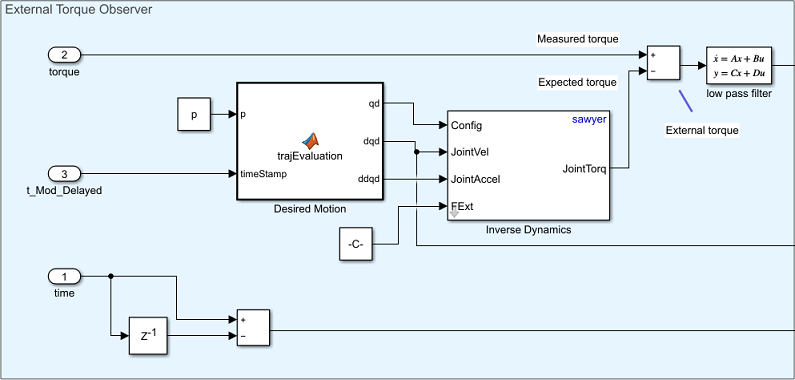In the Desired Motion block, the polynomial coefficients are given to generate the desired trajectory with the given `timeStamp` input, which can be adjusted based on the trajectory scaling algorithm. The ${q}_{d}\left(t\right)$, ${\underset{}{\overset{˙}{q}}}_{d}\left(t\right)$ and ${\underset{}{\overset{¨}{q}}}_{d}\left(t\right)$ are outputs based on the trajectory polynomial and are fed to the Computed Torque Controller subsystem.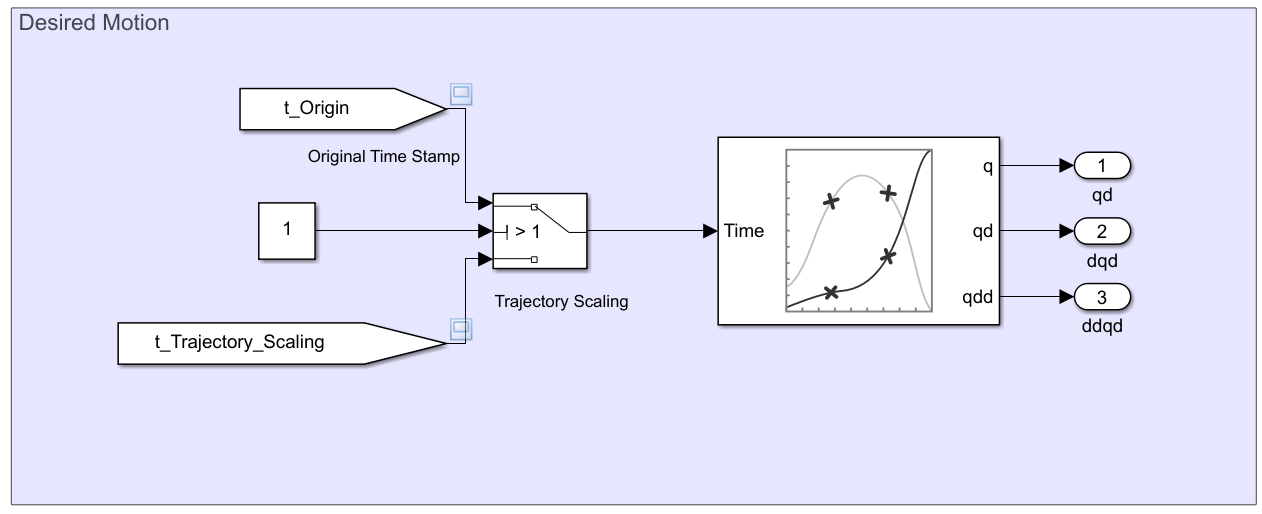### Simscape Multibody Robot Model and Simple Contact Mechanics

The Simscape Multibody Robot Model is imported from the same `.urdf` file using `smimport`, where a set of torque actuators and sensors for joint torque, joint position and velocity are added. A contact mechanism block as shown below is added to simulate the reaction force between the end effector and the obstacle as a sphere and a plane, where a simple linear spring-damper model is used.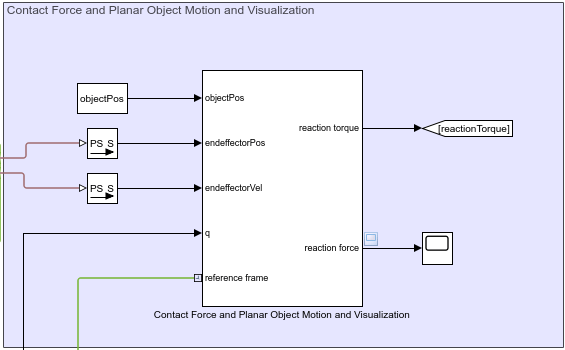Note: The contact mechanism has only been implemented between the end effector and the planar object. Therefore, other parts of the robot arm may still pass through the obstacle.

### Run the Model

Run the model and observe the behavior of Sawyer in the robot simulator and interact with it.

• First, open the viewer by clicking on the scope icon shown below on the left of the Simscape model block. It displays signals including the joint torques, reaction contact force between the end effector and the planar object, and the time stamp for calculating desired motion for trajectory tracking.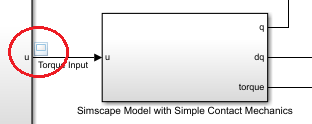• Toggle the trajectory scaling switch to "Off".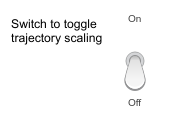• Click the Play button in Simulink to start the simulation. You should see the arm collide with the object yielding high torque inputs and a large reaction force. Note in this case the original time stamp is used. Stop the simulation afterwards.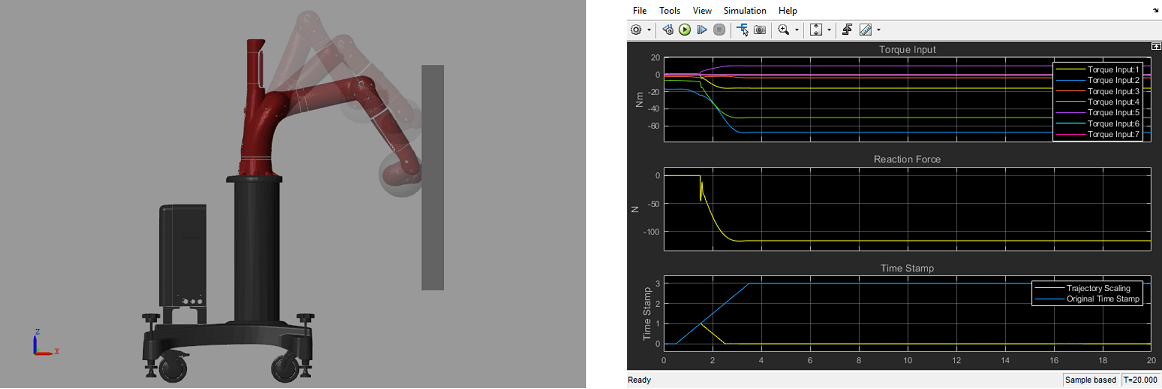• Now, toggle trajectory scaling switch to "On" and rerun the model. Notice the differences in the computed torques and the reduced reaction force after the collision.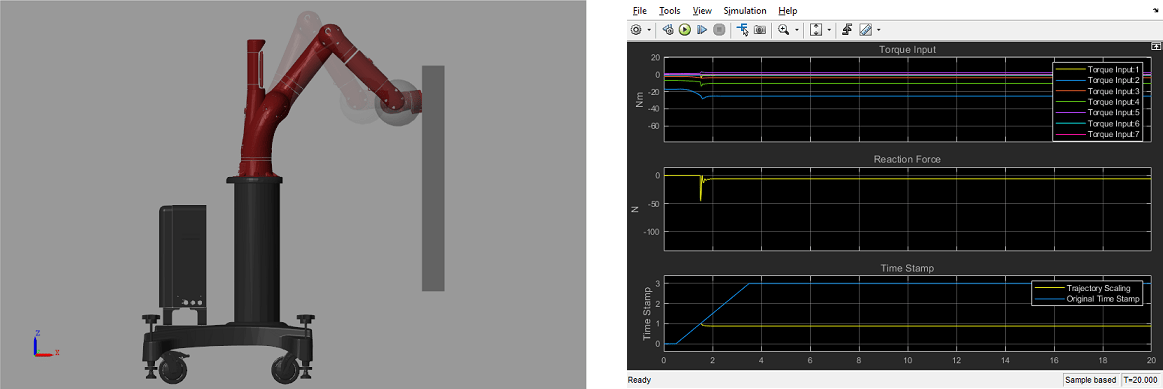• While the simulation is running, adjust the slider to move the object towards or away from the robot. The robot should react to its position while still trying to execute the trajectory safely.

• Click the Stop button in Simulink to stop the simulation.

### Summary

This example showed how to use robotics manipulator blocks in Simulink to design a computed torque controller, and integrate it with trajectory scaling and dynamic simulation in Simscape Multibody to achieve safe trajectory tracking. The resultant torque, reaction force and time stamp also demonstrated the capability of trajectory scaling for performing safe trajectory tracking.

##### SupportGet trial now